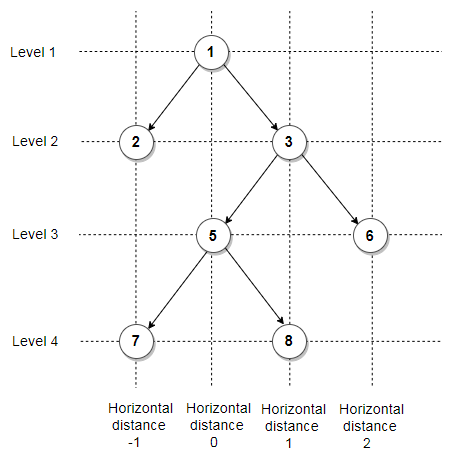# Print Bottom View of Binary Tree

Given an binary tree, print bottom view of it. Assume, the left and right child of a node makes 45 degree angle with the parent.

For example, Bottom view of below tree is 7, 5, 8, 6This problem can be easily solved with the help of Hashing. The idea is to create an empty map where each key represents the relative horizontal distance of the node from the root node and value in the map maintains a pair containing node’s value and its level number. Then we do a pre-order traversal of the tree and if current level of a node is more than or equal to maximum level seen so far for the same horizontal distance as current node’s or current horizontal distance is seen for the first time, we update the value and the level for current horizontal distance in the map. For each node, we recurse for its left subtree by decreasing horizontal distance and increasing level by 1 and recurse for right subtree by increasing both level and horizontal distance by 1.

Below figure shows horizontal distance and level of each node in above binary tree. The final values in the map will be

(horizontal distance -> (node’s value, node’s level))
-1 -> (7, 4)
0 -> (5, 3)
1 -> (8, 4)
2 -> (6, 3)## C++

The time complexity of above solution is O(nlog(n)) and auxiliary space used by the program is O(n).

Exercise: Modify the solution to print top view of binary tree(1 votes, average: 5.00 out of 5)Loading...

Please use our online compiler to post code in comments. To contribute, get in touch with us.
Like us? Please spread the word and help us grow. Happy coding 🙂